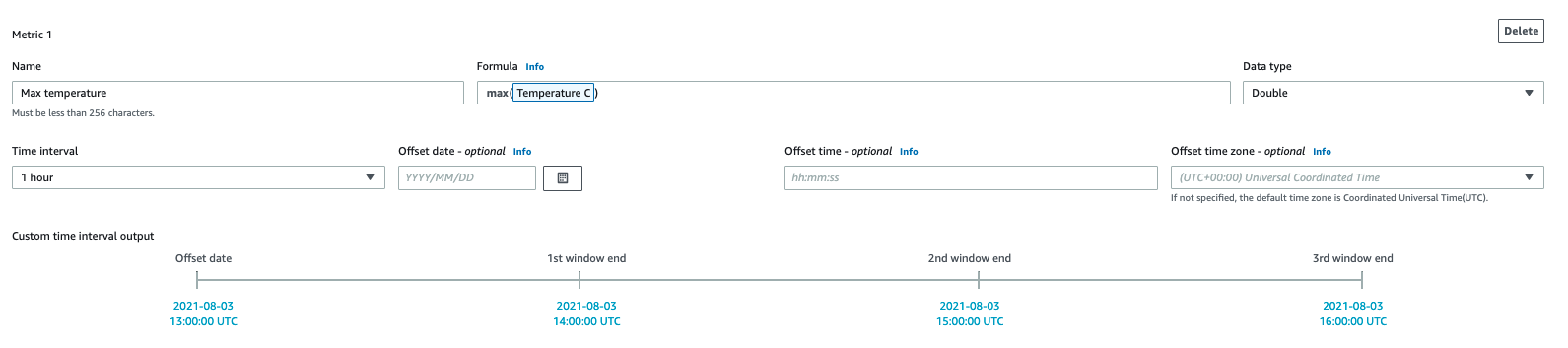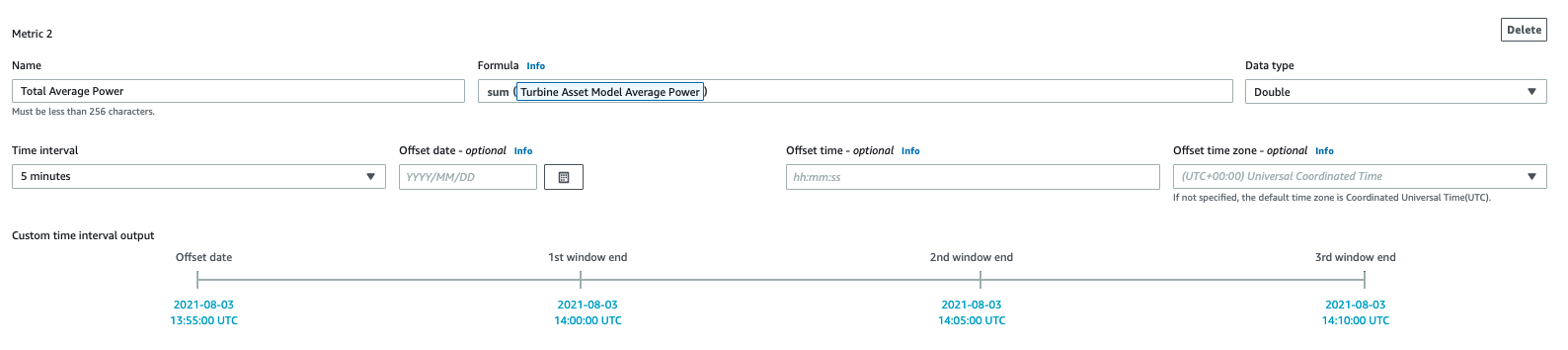Aggregating data from properties and other assets (metrics) - Amazon IoT SiteWise
Services or capabilities described in Amazon Web Services documentation might vary by Region. To see the differences applicable to the China Regions, see Getting Started with Amazon Web Services in China.

# Aggregating data from properties and other assets (metrics)

Metrics are mathematical expressions that use aggregation functions to process all input data points and output a single data point per specified time interval. For example, a metric can calculate the average hourly temperature from a temperature data stream.

Metrics can input data from associated assets' metrics, so you can calculate statistics that provide insight to your operation or a subset of your operation. For example, a metric can calculate the average hourly temperature across all wind turbines in a wind farm. For more information about how to define associations between assets, see Defining relationships between asset models (hierarchies).

Metrics can also input data from other properties without aggregating data over each time interval. If you specify an attribute in a formula, Amazon IoT SiteWise uses the latest value for that attribute when it computes the formula. If you specify a metric in a formula, Amazon IoT SiteWise uses the last value for the time interval over which it computes the formula. This means you can define metrics like ```OEE = Availability * Quality * Performance```, where `Availability`, `Quality`, and `Performance` are all other metrics on the same asset model.

Amazon IoT SiteWise also automatically computes a set of basic aggregation metrics for all asset properties. To reduce computation costs, you can use these aggregates instead of defining custom metrics for basic computations. For more information, see Querying asset property aggregates.

## Defining metrics (console)

When you define a metric for an asset model in the Amazon IoT SiteWise console, you specify the following parameters:

• Name – The property's name.

• Formula – The metric expression. Metric expressions can use aggregation functions to input data from a property for all associated assets in a hierarchy. Start typing or press the down arrow key to open the autocomplete feature. For more information, see Using formula expressions.

Important

Metrics can only be properties that are integer, double, Boolean, or string type. Booleans convert to `0` (false) and `1` (true).

If you define any metric input variables in a metric's expression, those inputs must have the same time interval as the output metric.

Formula expressions can only output double or string values. Nested expressions can output other data types, such as strings, but the formula as a whole must evaluate to a number or string. You can use the jp function to convert a string to a number. The Boolean value must be 1 (true) or 0 (false). For more information, see Undefined, infinite, and overflow values.

• Data type – The data type of the transform, which can be Double or String.

• Time interval – The metric time interval. Amazon IoT SiteWise supports the following tumbling window time intervals, where each interval starts when the previous one ends:

• 1 minute1 minute, computed at the end of each minute (12:00:00 AM, 12:01:00 AM, 12:02:00 AM, and so on).

• 5 minutes5 minutes, computed at the end of every five minutes starting on the hour (12:00:00 AM, 12:05:00 AM, 12:10:00 AM, and so on).

• 15 minutes15 minutes, computed at the end of every fifteen minutes starting on the hour (12:00:00 AM, 12:15:00 AM, 12:30:00 AM, and so on).

• 1 hour1 hour (60 minutes), computed at the end of every hour in UTC (12:00:00 AM, 01:00:00 AM, 02:00:00 AM, and so on).

• 1 day1 day (24 hours), computed at the end of every day in UTC (12:00:00 AM Monday, 12:00:00 AM Tuesday, and so on).

• 1 week1 week (7 days), computed at the end of every Sunday in UTC (every 12:00:00 AM Monday).

• Custom interval – You can enter any time interval between a minute and a week.

• Offset date – (Optional) The reference date from which to aggregate data.

• Offset time – (Optional) The reference time from which to aggregate data. The offset time must be between 00:00:00 and 23:59:59.

• Offset time zone – (Optional) The time zone for the offset. If it isn't specified, the default offset time zone is the Universal Coordinated Time (UTC).

• (UTC+00:00) Universal Coordinated Time

• (UTC+01:00) European Central Time

• (UTC+02:00) Eastern European

• (UTC03+:00) Eastern African Time

• (UTC+04:00) Near East Time

• (UTC+05:00) Pakistan Lahore Time

• (UTC+05:30) India Standard Time

• (UTC+07:00) Vietnam Standard Time

• (UTC+08:00) China Taipei Time

• (UTC+09:00) Japan Standard Time

• (UTC+09:30) Australia Central Time

• (UTC+10:00) Australia Eastern Time

• (UTC+11:00) Solomon Standard Time

• (UTC+12:00) New Zealand Standard Time

• (UTC-11:00) Midway Islands Time

• (UTC-10:00) Hawaii Standard Time

• (UTC-08:00) Pacific Standard Time

• (UTC-07:00) Phoenix Standard Time

• (UTC-06:00) Central Standard Time

• (UTC-05:00) Eastern Standard Time

• (UTC-04:00) Puerto Rico and US Virgin Islands Time

• (UTC-03:00) Argentina Standard Time

• (UTC-02:00) South Georgia Time

• (UTC-01:00) Central African Time

Example custom time interval with an offset (console)

The following example shows you how to define a 12-hour time interval with an offset on February 20, 2021, at 6:30:30 PM (PST).

To define a custom interval with an offset

1. For Time interval, choose Custom interval.

2. For Time interval, do one of the following:

• Enter `12`, and then choose hours.

• Enter `720`, and then choose minutes.

• Enter `43200`, and then choose seconds.

Important

The Time interval must be an integer regardless of the unit.

3. For Offset date, choose 2021/02/20.

4. For Offset time, enter `18:30:30`.

5. For Offset timezone, choose (UTC-08:00) Pacific Standard Time.

If you create the metric on July 1, 2021, before or at 06:30:30 PM (PST), you get the first aggregation result on July 1, 2021, at 06:30:30 PM (PST), the second aggregation result on July 2, 2021, at 06:30:30 AM (PST), and so on.

Example metric definition

The following example demonstrates a metric property that aggregates an asset's temperature data to calculate maximum hourly temperature.Example metric definition that inputs data from associated assets

The following example demonstrates a metric property that aggregates multiple wind turbines' average power data to calculate total average power for a wind farm.## Defining metrics (CLI)

When you define a metric for an asset model with the Amazon IoT SiteWise API, you specify the following parameters:

• `name` – The property's name.

• `dataType` – The data type of the metric, which can be `DOUBLE` or `STRING`.

• `expression` – The metric expression. Metric expressions can use aggregation functions to input data from a property for all associated assets in a hierarchy. For more information, see Using formula expressions.

• `window` – The time interval and offset for the metric's tumbling window, where each interval starts when the previous one ends:

• `interval` – The time interval for the tumbling window. The time interval must be between a minute and a week.

• `offsets` – The offset for the tumbling window.

For more information, see TumblingWindow in the Amazon IoT SiteWise API Reference.

Example custom time interval with an offset (Amazon CLI)

The following example shows you how to define a 12-hour time interval with an offset on February 20, 2021, at 06:30:30 PM (PST).

``````{
"window": {
"tumbling": {
"interval": "12h",
"offset": " 2021-07-23T18:30:30-08"
}
}
}``````

If you create the metric on July 1, 2021, before or at 06:30:30 PM (PST), you get the first aggregation result on July 1, 2021, at 06:30:30 PM (PST), the second aggregation result on July 2, 2021, at 06:30:30 AM (PST), and so on.

• `variables` – The list of variables that defines the other properties of your asset or child assets to use in the expression. Each variable structure contains a simple name for use in the expression and a `value` structure that identifies which property to link to that variable. The `value` structure contains the following information:

• `propertyId` – The ID of the property from which to pull values. You can use the property's name instead of its ID if the property is defined in the current model (rather than defined in a model from a hierarchy).

• `hierarchyId` – (Optional) The ID of the hierarchy from which to query child assets for the property. You can use the hierarchy definition's name instead of its ID. If you omit this value, Amazon IoT SiteWise finds the property in the current model.

Important

Metrics can only be properties that are integer, double, Boolean, or string type. Booleans convert to `0` (false) and `1` (true).

If you define any metric input variables in a metric's expression, those inputs must have the same time interval as the output metric.

Formula expressions can only output double or string values. Nested expressions can output other data types, such as strings, but the formula as a whole must evaluate to a number or string. You can use the jp function to convert a string to a number. The Boolean value must be 1 (true) or 0 (false). For more information, see Undefined, infinite, and overflow values.

• `unit` – (Optional) The scientific unit for the property, such as mm or Celsius.

Example metric definition

The following example demonstrates a metric property that aggregates an asset's temperature measurement data to calculate maximum hourly temperature in Fahrenheit. This object is an example of an AssetModelProperty that contains a Metric. You can specify this object as a part of the CreateAssetModel request payload to create a metric property. For more information, see Creating an asset model (CLI).

``````{
...
"assetModelProperties": [
...
{
"name": "Max temperature",
"dataType": "DOUBLE",
"type": {
"metric": {
"expression": "max(temp_f)",
"variables": [
{
"name": "temp_f",
"value": {
"propertyId": "Temperature F"
}
}
],
"window": {
"tumbling": {
"interval": "1h"
}
}
}
},
"unit": "Fahrenheit"
}
],
...
}``````

Example metric definition that inputs data from associated assets

The following example demonstrates a metric property that aggregates multiple wind turbines' average power data to calculate total average power for a wind farm. This object is an example of an AssetModelProperty that contains a Metric. You can specify this object as a part of the CreateAssetModel request payload to create a metric property. For more information, see Creating an asset model (CLI).

``````{
...
"assetModelProperties": [
...
{
"name": "Total Average Power",
"dataType": "DOUBLE",
"type": {
"metric": {
"expression": "avg(power)",
"variables": [
{
"name": "power",
"value": {
"propertyId": "a1b2c3d4-5678-90ab-cdef-11111EXAMPLE",
"hierarchyId": "Turbine Asset Model"
}
}
],
"window": {
"tumbling": {
"interval": "5m"
}
}
}
},
"unit": "kWh"
}
],
...
}``````